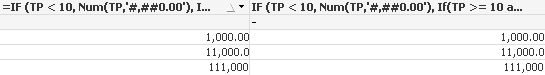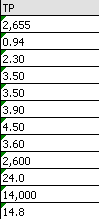# QlikView App Dev

Discussion Board for collaboration related to QlikView App Development.

Announcements
Become an analytics expert with Qlik's new 15 week course: Applied Data Analytics using Qlik Sense. READ MORE
cancel
Showing results for
Did you mean:Creator

## Excel Export - Numbers as Text

Dear Community members -

I've a load field called TP which is calculated this way in the script -

IF (TP < 10, Num(TP,'#,##0.00'), If(TP >= 10 and TP < 100, Num(TP,'#,##0.0'), Num(TP,'#,##0'))) as TP

Condition is -

TP < 10 - 2 decimal points,

TP >=10 and TP<100 - 1 decimal point,

Else TP has no decimal point.

Then I’m using this field in a straight table but when I export it to excel it exports in ‘number converted as text’ format in excel.

I’ve seen many posts and tried many solutions nothing helped me. If the field didn’t have the above mentioned condition then it’s exporting in correct format.

Any help will be appreciated.

1 Solution

Accepted SolutionsCreator
Author

I solved this by using round function in an expression. It doesn't solve my problem fully but serves the purpose for now.

Anybody gets any idea on this do reply on this post. I'm closing this discussion.

Cheers!

5 RepliesAnonymous
Not applicable

I Format such an Expression as calculated Dimension or as Expression (whereever you want it)

if Expression you may Need an Expression like sum et.c

Image was just as example (left is calculated Dimension, right isExpression)

IF (TP < 10, Num(TP,'#,##0.00'), If(TP >= 10 and TP < 100, Num(TP,'#,##0.0'), Num(TP,'#,##0')))Creator
Author

Hi Rudolf

Thanks for that

but I've already tried this. It still exports as 'numbers stored as text'Creator II

Try Round function

=Round(86.656982, 0.01)

=Round(86.656982, 0.1)

=Round(86.656982, 0)

Hope this will help !!Creator
Author

I tried this as a calculated dimension but didn't work.

 =If(TP < 10, Round(TP,0.01), If(TP >= 10 and TP < 100, Round(TP,0.1), Round(TP,0))) //TP - 0.94 2.3 3.5 3.9 3.5 4.5 -Creator
Author

I solved this by using round function in an expression. It doesn't solve my problem fully but serves the purpose for now.

Anybody gets any idea on this do reply on this post. I'm closing this discussion.